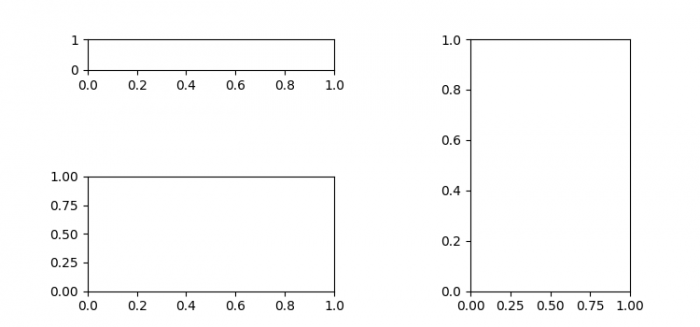# Adjusting the heights of individual subplots in Matplotlib in Python

To adjust the heights of individual subplots in Matplotlib in Python, we can take the following steps −

• Set the figure size and adjust the padding between and around the subplots.
• Add a subplot to the current figure, nrows=7, ncols=2 and at index=1.
• Add a subplot to the current figure, nrows=2, ncols=2 and at index=3
• Add a subplot to the current figure, nrows=1, ncols=3 and at index=3
• To display the figure, use show() method.

## Example

import matplotlib.pyplot as plt

plt.rcParams["figure.figsize"] = [7.50, 3.50]
plt.rcParams["figure.autolayout"] = True

plt.subplot(7, 2, 1)
plt.subplot(2, 2, 3)
plt.subplot(1, 3, 3)

plt.show()

## Output